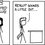# And you thought limits were always easy

Another CMI problem: (and I could not solve this)

Consider a continuous function $f : [-1,1] \rightarrow R$ which is differentiable at $0$. Prove that the following limit exists:

$\displaystyle \lim_{r \to 0^{+}} \left( \int_{-1}^{-r} \dfrac{ f(x) } {x } dx + \int_{r}^1 \dfrac{ f(x) } {x } dx \right)$

Also give an example of a function $f$ to show that the limit need not exist if it is not differentiable at $0$.

In case there is a mistake in the question (I don't think there should be), please tell me. The problem is, I don't have the question paper and it hasn't yet been uploaded on their site. So if there is any error, it must have crept in due to my poor memory.Note by Parth Thakkar
7 years, 2 months ago

This discussion board is a place to discuss our Daily Challenges and the math and science related to those challenges. Explanations are more than just a solution — they should explain the steps and thinking strategies that you used to obtain the solution. Comments should further the discussion of math and science.

When posting on Brilliant:

• Use the emojis to react to an explanation, whether you're congratulating a job well done , or just really confused .
• Ask specific questions about the challenge or the steps in somebody's explanation. Well-posed questions can add a lot to the discussion, but posting "I don't understand!" doesn't help anyone.
• Try to contribute something new to the discussion, whether it is an extension, generalization or other idea related to the challenge.

MarkdownAppears as
*italics* or _italics_ italics
**bold** or __bold__ bold
- bulleted- list
• bulleted
• list
1. numbered2. list
1. numbered
2. list
Note: you must add a full line of space before and after lists for them to show up correctly
paragraph 1paragraph 2

paragraph 1

paragraph 2

[example link](https://brilliant.org)example link
> This is a quote
This is a quote
    # I indented these lines
# 4 spaces, and now they show
# up as a code block.

print "hello world"
# I indented these lines
# 4 spaces, and now they show
# up as a code block.

print "hello world"
MathAppears as
Remember to wrap math in $$ ... $$ or $ ... $ to ensure proper formatting.
2 \times 3 $2 \times 3$
2^{34} $2^{34}$
a_{i-1} $a_{i-1}$
\frac{2}{3} $\frac{2}{3}$
\sqrt{2} $\sqrt{2}$
\sum_{i=1}^3 $\sum_{i=1}^3$
\sin \theta $\sin \theta$
\boxed{123} $\boxed{123}$

Sort by:

One thing that i can interpret from this question is that if f(x) is not differentiable at x=0 then f(x)/x will not be continuous at x=0 i.e. the right hand side and left hand side of 0 will attain different values and which makes the limit not to exist for f(x)

- 7 years, 2 months ago

Why should it be true, that "if $f(x)$ is not differentiable at $x = 0$, then $\dfrac{f(x)}{x}$ will not be continuous at $x = 0$."? I haven't found a counter example, but could you please give a reason?

$\\$

Also, regarding your second clain: Let's consider $f(x) = |x|$. Clearly, this isn't differentiable at $x = 0$, and also, $\dfrac{f(x)}{x}$ isn't continuous at $x = 0$. (Agrees with your first claim).

$\\$

Now, the integrals $\displaystyle \int_{-1}^{-r} \dfrac{ f(x) } {x } dx$ and $\displaystyle \int^{1}_{+r} \dfrac{ f(x) } {x } dx$ will equal $-1 + r$ and $1 - r$ respectively. So, the limit is $-1 + 1 = 0$. Doesn't it exist?

$\\$

Obviously, this doesn't answer the question - which is, given a differentiable function show that the limit exists, while my example shows that there exists a non-differentiable function such that the limit exists. But this does counter your second claim ( "the right hand side and left hand side of $0$ will attain different values which makes the limit not to exist for $f(x)$"). I don't say that the right and and left hand sides are the same, but still, the limit does exist.

If there's something wrong, I'd love to see it! :)

- 7 years, 2 months ago

Other problems I found interesting:

- 7 years, 2 months ago

@Calvin Lin , could you help me out with this one? Thanks.

- 7 years, 2 months ago

with that i think the limit will always exist since the integral can always be broken into two different integrals about a point where its not continuous ... !! since r tends to 0+ and if we want to prove that the limit doesn't exists, the expression in the numerator should be a polynomial of degree less than that of in the denominator .. !!

- 7 years, 2 months ago